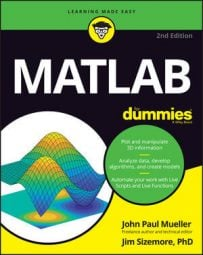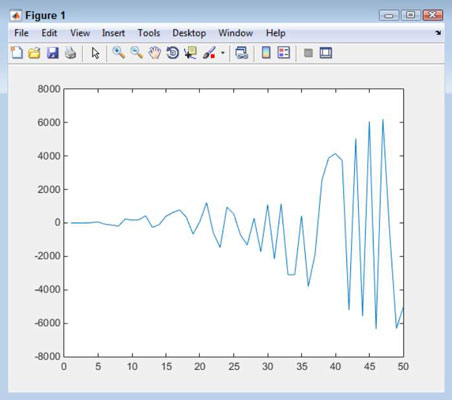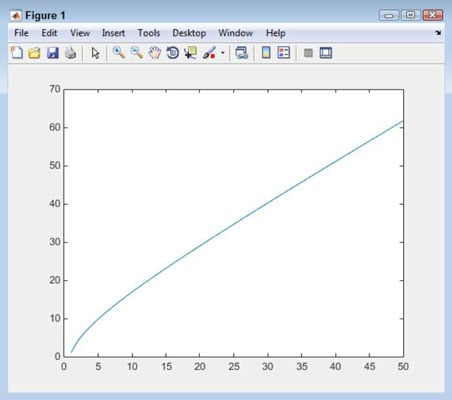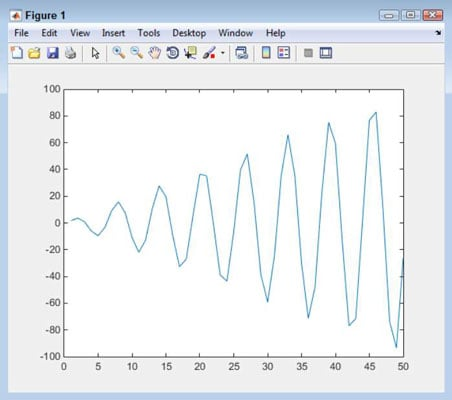##### MATLAB For DummiesYou can use MATLAB with the Symbolic Math Toolbox to solve a number of simple calculus problems. Calculus can solve myriad problems that algebra can’t. It’s really the study of how things change. This branch of math is essentially split into two pieces: differential calculus, which considers rates of change and slopes of curves, and integral calculus, which considers the accumulation of quantities and the areas between and under curves.

## Working with differential calculus

MATLAB offers good differential calculus support. This example starts with something simple: Univariate differentiation. (Remember that univariate differentiation has a single variable.) MATLAB supports a number of forms of differential calculus — each of which requires its own set of functions. In this case, you use the diff() function to perform the required tasks. The following steps help you perform a simple calculation:

1. Type syms x and press Enter.

MATLAB creates a symbolic object to use in the calculation.

2. Type f(x) = sin(x^3) and press Enter.

Doing so creates the symbolic function used to perform the calculation. Here’s the output you see:

```f(x) =
sin(x^3)```
3. Type Result = diff(f) and press Enter.

The output shows the result of the differentiation:

```Result(x) =
3*x^2*cos(x^3)```

Result(x) is actually a symbolic function. You can use it to create a picture of the output.

4. Type plot(Result(1:50)) and press Enter.## Using integral calculus

You’ll also find great integral calculus support in MATLAB. This example focuses on a univariate calculation. In this case, the example relies on the int() function to perform the required work. The following steps help you perform a simple calculation:

1. Type syms x and press Enter.

MATLAB creates a symbolic object to use in the calculation.

2. Type f(x) = (x^3 + 3*x^2) / x^3 and press Enter.

The symbolic function that you create produces the following output:

```f(x) =
(x^3 + 3*x^2)/x^3```
3. Type Result = int(f, x) and press Enter.

Notice that you must provide a symbolic variable as the second input. The output shows the following symbolic function as the result of the integration:

```Result(x) =
x + 3*log(x)```
4. Type plot(Result(1:50)) and press Enter.## Working with multivariate calculus

Many (if not most) problems don’t involve just one variable. With this in mind, the following steps demonstrate a problem with more than one variable — a multivariate example:

1. Type syms x y and press Enter.

MATLAB creates the two symbolic objects used for this calculation.

2. Type f(x, y) = x^2 * sin(y) and press Enter.

This symbolic function accepts two inputs, x and y, and uses them to perform a calculation. Here’s the output from this step:

```f(x, y) =
x^2*sin(y)```
3. Type Result = diff(f) and press Enter.

The output shows the result of the differentiation:

```Result(x, y) =
2*x*sin(y)```

In this case, Result(x, y) accepts two inputs, x and y. As before, you can create a picture from the output of Result().

The example shows the derivative with respect to x, which is the default. To obtain the derivative with respect to y (df/dy), you type diff(f, y) instead.

4. Type plot(Result(1:50, 1:50)) and press Enter.

Notice that in this case, you must provide both x and y inputs, which isn’t surprising. However, the two vectors must have the same number of elements or MATLAB will raise an exception.